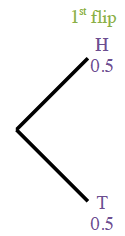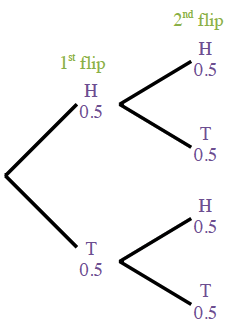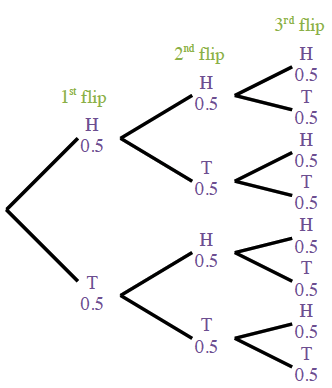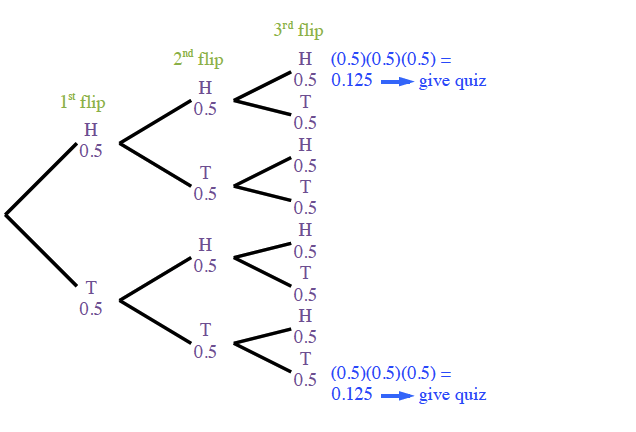### Home > GC > Chapter 6 > Lesson 6.2.2 > Problem6-55

6-55.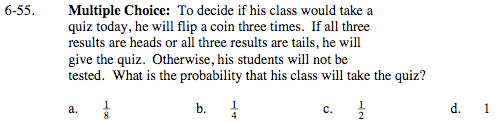Use a tree diagram to calculate the probability.

For help with the probability models, see Lesson 4.2.4.

Draw the tree diagram one step at a time.

Calculate the probability of three consecutive heads (H) or tails (T).

If you follow the branches of the tree diagram for H, H, H you get the P(H,H,H) by multiplying (0.5)(0.5)(0.5) = 0.125 --> give quiz

If you follow the branches of the tree diagram for T, T, T you get the P(T,T,T) by multiplying (0.5)(0.5)(0.5) = 0.125 --> give quiz

Calculate the probability that the teacher will give the quiz. To do so add the P(H,H,H) + P(T,T,T).

P(give quiz) = 0.125 + 0.125 = 0.25.

B; see response in Step 5 for reason.